# 2nd PUC Physics Question Bank Chapter 8 Electromagnetic Waves

## Karnataka 2nd PUC Physics Question Bank Chapter 8 Electromagnetic Waves

### 2nd PUC Physics Electromagnetic Waves NCERT Text Book Questions and Answers

Question 1.
Figure 8.6 shows a capacitor made of two circular plates each of radius 12 cm, and separated by 5.0 cm. The capacitor is being charged by an external source (not shown in the figure). The charging current is constant and equal to 0.15A.
(a) Calculate the capacitance and the rate of charge of potential difference between the plates.
(b) Obtain the displacement current across the plates.
(c) Is Kirchhoff’s first rule (junction rule) valid at each plate of the capacitor? Explain.(c) Yes, Kirchhoff’s first law will be valid at each plate of the capacitor, provided the electric current means the sum of the conduction and the displacement currents.

Question 2.
A parallel plate capacitor (Fig. 8.7) made of circular plates each of radius R = 6.0 cm has a capacitance C = 100 pF. The capacitor is connected to a 230 Y ac supply with an (angular) frequency of 300 rad s1.
(a) What is the rms value of the conduction current?
(b) Is the conduction current equal to the displacement current?
(c) Determine the amplitude of B at a point 0 cm from the axis between the plates.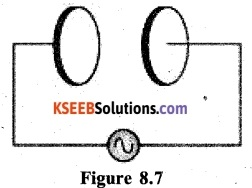(b) Yes the conduction current and the displacement current will be equal. It is true, even if the conduction current is oscillating.
(c) Let us first derive an expression for the amplitude of the magnetic field at a distance x from the axis of the capacitor. To do so, place a circular loop of radius r between the two plates of the capacitor with its face parallel to the plates and its centre on the axis of the plates.
According to modified Ampere’s circuital law,The amplitude of the magnetic field at a distance r from the axis of the plates is then, given by.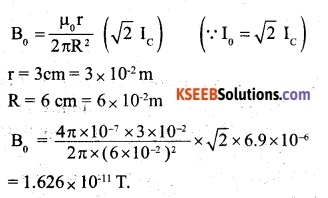Question 3.
What physical quantity is the same for X- rays of wavelength 10-1 m, the red light of wavelength 6800 A, and radio waves of wavelength 500m?
All e.m. waves travel with the same speed c = 3 x 108 m s-1 in a vacuum.
Therefore, X-rays, red light, and radio waves have the same speed.

Question 4.
A plane electromagnetic wave travels in a vacuum along the z-direction. What can you say about the directions of its electric and magnetic field vectors? If the frequency of the wave is 30 MHz, what is its wavelength?
The electric and magnetic field vectors are in X Y – plane,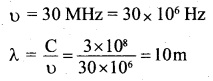Question 5.
A radio can tune in to any station in the 7.5 MHz to 12 MHz bands. What is the corresponding wavelength band?
Corresponding to v = 7.5 MHz = 7.5 × 106HzQuestion 6.
A charged particle oscillates about its mean equilibrium position with a frequency of 109. What is the frequency of the electromagnetic waves produced by the oscillator?
The frequency of the produced E.M. wave is the same as the frequency of oscillating charged particles i. e. 109 Hz.Question 7.
The amplitude of the magnetic field part of a harmonic electromagnetic wave in a vacuum is Bo = 510 nT. What is the amplitude of the electric field part of the wave?
B0 = 510 nT = 510 x 10-9T
The amplitude of the electric field part of the wave,
E0 =CB0 =3 x l08 x 510 x l0-9 =153NC-4

Question 8.
Suppose that the electric field amplitude of an electromagnetic wave is E0 = 120 N/ C and that its frequency is v = 50.0 MHz.
(a) Determine, B0,ω, k, and λ,
(b) Find expressions for E and B.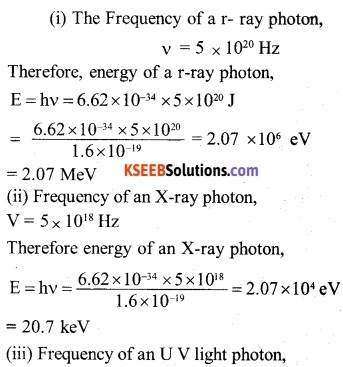Question 9.
The terminology of different parts of the electromagnetic spectrum is given in the text. Use the formula E = hv (for energy of a quantum of radiation: photon) and obtain the photon energy in units of eV for different parts of the electromagnetic spectrum. In what way are the different scales of photon energies that you obtain related to the sources of electromagnetic radiation?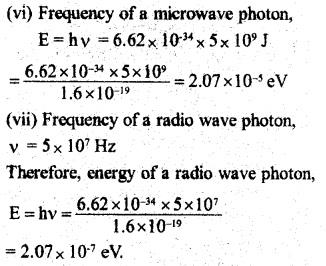Question 10.
In a plane electromagnetic wave, the electric field oscillates sinusoidally at a frequency of 2.0 x 1010 Hz and amplitude 48 V m-1.
(a) What is the wavelength of the wave?
(b) What is the amplitude of the oscillating magnetic field?
(c) Show that the average energy density of the E field equals the average energy density of the B field, [c = 3 x 108 ms-1]
u =2 x 1010Hz
E0 = 48 Vm4 C
= 3 x l08ms-1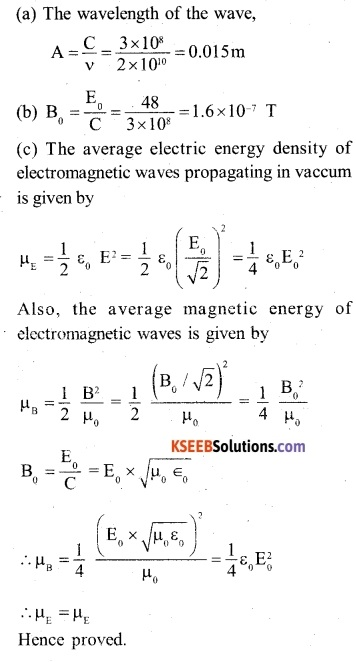Question 11.
Suppose that the electric field part of an electromagnetic wave in a vacuum is E= {(3.1 N/C) cos [(1.8 rad/m) y + (5.4 x 106 rad/s)t]} $$\hat { i }$$

• What is the direction of propagation?
• What is the wavelength λ?
• What is the frequency V?
• What is the amplitude of the magnetic field part of the wave?
• Write an expression for the magnetic field part of the wave.

The electric field part of the electromagnetic wave is given by,
$$\vec { E }$$ = (3.1 NC-1 ) cos [(1,8 rad m-1) y + (5.4x 106 rad s1) t]$$\hat { i }$$ it follows that
E0 = 3.1 NC-1
(a) Since the argument of since in the expression for electric field is of type (ky +cot), the direction of propagation of the electromagnetic wave is along the negative Y-axis.

(b) The wavelength of the wave,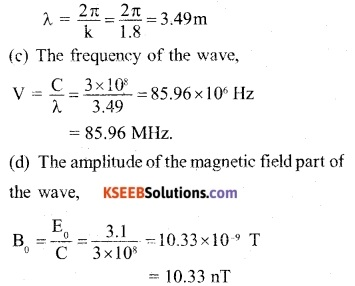(e) The electric field and the direction of propagation of the e.m. wave are along negative X-axis and negative Y-axis respectively. Therefore, the magnetic field is along negative Z-axis and expression for it is given by
B = (10.33nT) cos [(1.8 rad m-1) y + (5.4 x 106rad s-1)t] $$\hat { k }$$Question 12.
About 5% of the power of a 100 W light bulb is converted to visible radiation. What is the average intensity of visible radiation
(a) At a distance of lm from the bulb?
(b) At a distance of 10 m? Assume that the radiation is emitted isotropically and neglect reflection.
P = 100 W
n = 5%
Since the efficiency of the bulb is 5%, the effective power of the bulb.
$${ P }_{ eff }=p\times n=100\times \frac { 5 }{ 100 } =5w$$
At a distance, ‘r’ the radiation coming from the bulb is distributed over the surface of a sphere of radius r i.e. over an area.
A = 4πr2
Therefore, the intensity of light at a distance of r,Question 13.
Use the formula λmT = 29 cm K to obtain the characteristic temperature ranges for different parts of the electromagnetic spectrum. What do the numbers that you obtain tell you?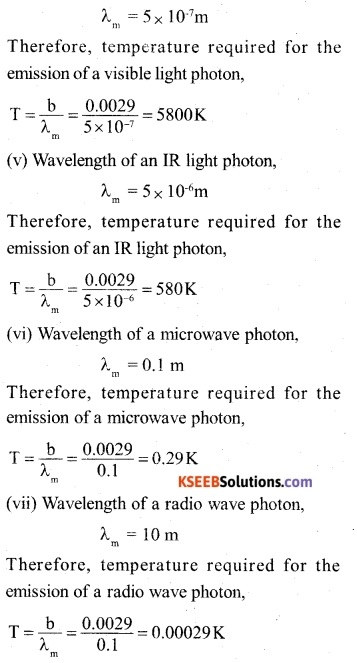These numbers tell us the order of temperature for obtaining radiations in different parts of the electromagnetic spectrum. At these temperatures, the radiation of the corresponding wavelength in a particular case can be emitted at a lower temperature also, but its intensity will not be maximum.

Question 14.
Given below are some famous numbers associated with electromagnetic radiations in different contexts in physics. State the part of the electromagnetic spectrum to which each belongs.
(a) 21 cm (wavelength emitted by atomic hydrogen in interstellar space).
(b) 1057 MHz (frequency of radiation arising from two close energy levels in hydrogen; known as Lamb shift).
(c) 7 K [temperature associated with the isotropic radiation fHifag nil space-thought to be a relic of the big-bang origin of the universe}.
(d) 5890 A – 5890 A (double lines of sodium}
(e) 14.4 keV {energy of a particular transition in 57Fe nucleus associated with a famous high-resolution spectroscopic method (Mossbauer spectroscopy)].
(a) Given wavelength is of the order of 10-2 m i.e. short radio wave.
(b) Frequency is of the order of 109 Hz e. short radio wave.
(c) Microwave
(d) Visible(Yellow)
(e) X-rays (or soft γ-rays) region

Question 15.

2. It is necessary to use. satellites for long-distance TV transmission. Why?
3. Optical and radio telescopes are built on the ground but X-ray astronomy is possible only from satellites orbiting the earth. Why?
4. The small ozone layer on top of the stratosphere is crucial for human survival. Why?
5. If the earth did not have an atmosphere, would its average surface temperature be higher or lower than what it is now?
6. Some scientists have predicted that a global nuclear war on the earth would be followed by a severe nuclear winter’ with a devastating effect on life on earth. What might be the basis of this prediction?

1. Long-distance radio broadcasts make use of sky waves. The ionosphere of the earth’s atmosphere reflects the radiations of this range.
2. For very long-distance TV transmission, a very high frequency is required. Waves of this frequency just pass through the ionosphere and are not reflected back. Therefore, a satellite is required to reflect the signals to the earth.
3. The atmosphere of the earth can absorb X-rays but visible waves and radio waves pass through it.
4. The ozone layer absorbs ultraviolet (UV) radiation emitted by the sun and prevents them to reach the earth. Ultraviolet radiations are harmful for the life on earth.
5. In this case, there will be no greenhouse effect. So the earth will be at a low temperature in the absence of the atmosphere.
6. In the case of worldwide nuclear war, the sky may get overcast with clouds of nuclear radiation. These clouds will stop the passage of sunlight to earth. Thus, the earth will be as cool as in winter.

Question 1.
What is Maxwell’s displacement current?
The displacement current is produced, when in an electric circuit (say across the plates of a charged capacitor), the electric field changes with time. Mathematically,Question 2.
Can an electromagnetic wave be deflected by a magnetic or electric field? Explain.
No, an electromagnetic wave cannot be deflected by a magnetic or electric field. It is because they do not consist of charged particles. They merely propagate in the form of electric and magnetic fields varying both in space and time.

Question 3.
The dimensions of $$\frac { 1 }{ { \mu }_{ 0 }{ \in }_{ 0 } }$$ where symbols have their usual meanings are:
(A) [L1 T]
(B) [L2 T2]
(C) [L2 T-2]
(D) [L T1]
The velocity of the electromagnetic wave in free spaceQuestion 4.
Which of the following are not electromagnetic waves
(A) Cosmic rays
(B) γ-rays
(C) β – rays
(D) X-rays
(C) β – rays

Question 5.
If $${ \in }_{ 0 }$$ and $${ \mu }_{ 0 }$$ are the electric permeability and magnetic permeability in free space, ∈ and µ are the corresponding quantities in a medium, then index of refraction of the medium is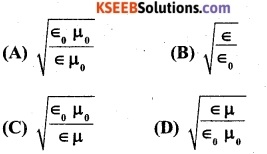The velocity of the electromagnetic waves in free space is given by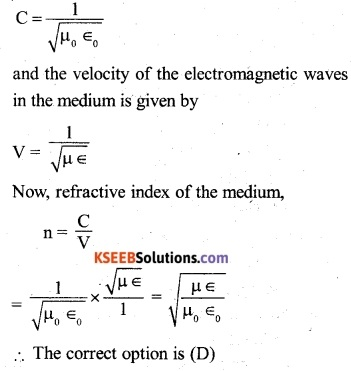Question 6.
The speed of electromagnetic waves is independent of
(A) Wavelength
(B) Frequencies
(C) Intensity
(D) Medium in which it travels
(C) IntensityQuestion 7.
If an electromagnetic wave propagating through vacuum is described by,
E = E0 sin (Kx – wt); B = B0 sin (Kx -ωt) then,
(A) E0K = Boω
(B) E0B0 = ωK
(C) E0ω=B0K
(D) E0B0=ω/K
(A) E0K = Boω

Question 8.
The oscillating magnetic field in a plane electromagnetic wave is given by
By = 8×106sin [2 × 10-6t + 300 πx] (int) calculate the wavelength of the wave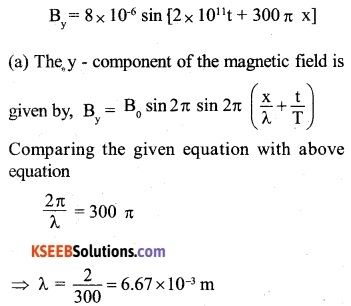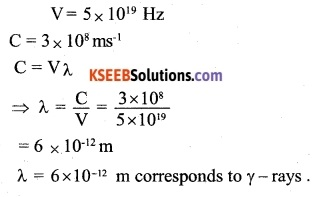The velocity of light rays of different wavelengths in a vacuum is the same and hence the ratio of their velocities is 1.